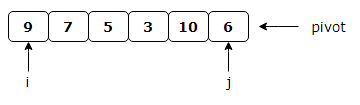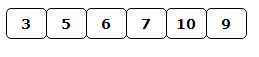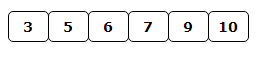# Quick Sort Algorithm In C#

## Introduction

In this article, I am going to explain about the Quicksort algorithm.This is a Divide and Conquer algorithm. It picks an element as pivot and partitions the given array around the picked pivot.

This algorithm is very common in job interviews.So first, I am going to explain Quick Sort algorithm; then, I will be providing the C# code to execute it.

## The Quicksort Algorithm

This Algorithm selects an element as pivot element from the given array and partitions the array around it such that, Left side of pivot contains all the elements that are less than the pivot element. The right side contains all elements that are greater than the pivot element. Let P be the index of pivot after partitioning the array. Then, the left subarray(start to P-1) and right subarray(P+1 to end) are sorted recursively to get the final sorted array as output.

Different versions of Quicksort pick pivot in different ways such as.

1. Always pick the first element as a pivot.
2. Always pick the last element as pivot
3. Pick a random element as pivot.
4. Pick median as pivot.

Selecting a pivot element reduces the space complexity and removes the use of the auxiliary array that is used in merge sort. Selecting a random pivot in an array results in an improved time complexity in most of the cases.

## How the array is partitioned around pivot?

1. Assume the last element as pivot .
2. We want larger elements to go to the right and smaller to go to left. We maintain two indexes i and j, whereas i pointing to the leftmost element and j is pointing to last element(pivot).
3. While i is to the left of j, we move i right, skipping all the elements less than the pivot. If an element is found greater than the pivot, i stops
4. While j is to the right of i, we move j left, skipping all the elements greater than the pivot. If an element is found less than the pivot, j stops
5. When both i and j have stopped, the elements are swapped.
6. When i and j have crossed, no swap is performed, scanning stops, and the element pointed to by i is swapped with the pivot.

Let us understand this with the help of an example.

Let us take input array as – 9 7 5 3 10 6

The sorted output for this array is – 3 5 6 7 9 10

### Step 1

Select the last element (6) as pivot element and partition the array around 6.### Step 2

After partitioning, we get the following.### Step 3

Now, we will recursively sort the left subarray (5 3).Which gives –### Step 4

Since the left subarray is now sorted, we will recursively sort the right subarray (7 10 9). The last element of right subarray (9) is selected as pivot and right subarray is partitioned around it. Which gives### Step 5

Since the left side of Pivot element(9) has only one element i.e (7) hence it is sorted. Similarly, the right side of pivot has only one element i.e (10) hence that is also sorted.

Hence, we get our sorted array using Quicksort as follows –## Time Complexity

The complexity of QuickSort depends on the selection of pivot.

The worst case time complexity of this algorithm is O(N²),if largest or smallest element is selected as pivot.

The best case occurs when the partition process always selects the middle element as pivot.This can be achieved by using random function to select pivot element.There are logN partitions, and to obtain each partitions we do N comparisons. Hence the complexity is O(NlogN).

The average case time complexity of this algorithm is also O(NLogN).

## QuickSort Vs MergeSort

Quicksort in its general form is an in-place sort (i.e. it doesn’t require any extra storage) whereas merge sort requires O(N) extra storage, N denoting the array size which may be quite expensive. Allocating and deallocating the extra space used for merge sort increases the running time of the algorithm. Comparing average complexity we find that both type of sorts have O(NlogN) average complexity. For arrays, merge sort loses due to the use of extra O(N) storage space.

In case of linked lists the case is different mainly due to difference in memory allocation of arrays and linked lists. Unlike arrays, linked list nodes may not be adjacent in memory. In linked list, we can insert items in the middle in O(1) extra space and O(1) time. Therefore merge operation of merge sort can be implemented without extra space for linked lists.